How Cheenta works to ensure student success?
Explore the Back-Story

# Test of Mathematics Solution Subjective 170 - Infinite Circles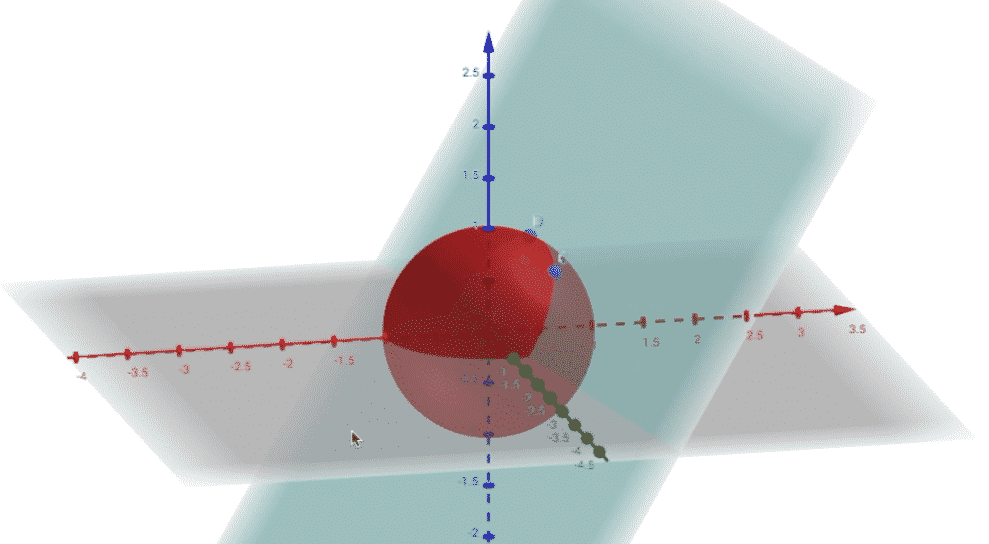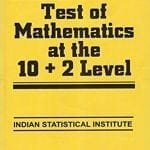This is a Test of Mathematics Solution Subjective 170 (from ISI Entrance). The book, Test of Mathematics at 10+2 Level is Published by East West Press. This problem book is indispensable for the preparation of I.S.I. B.Stat and B.Math Entrance.

Also visit: I.S.I. & C.M.I. Entrance Course of Cheenta

## Problem

Let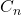be an infinite sequence of circles lying in the positive quadrant of the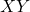-plane, with strictly decreasing radii and satisfying the following conditions. Eachtouches both the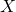-axis and the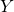-axis. Further, for all, the circle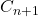touches the circleexternally. If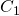has radius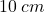, then show that the sum of the areas of all these circles is.

## Solution

Consider the following diagram where the Green line segment is, the radius of the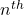circle, and the Yellow line segment is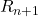.As we are told about the symmetricity of the figure in the problem we can say that: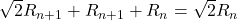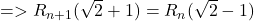Let's say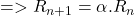.

Now the total sum of the areas of the circles is: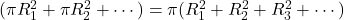Now as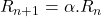, we can say that: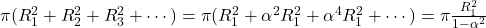as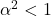.

Substituting the value of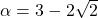and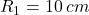we have,

Sum =.

Hence Proved.This is a Test of Mathematics Solution Subjective 170 (from ISI Entrance). The book, Test of Mathematics at 10+2 Level is Published by East West Press. This problem book is indispensable for the preparation of I.S.I. B.Stat and B.Math Entrance.

Also visit: I.S.I. & C.M.I. Entrance Course of Cheenta

## Problem

Letbe an infinite sequence of circles lying in the positive quadrant of the-plane, with strictly decreasing radii and satisfying the following conditions. Eachtouches both the-axis and the-axis. Further, for all, the circletouches the circleexternally. Ifhas radius, then show that the sum of the areas of all these circles is.

## Solution

Consider the following diagram where the Green line segment is, the radius of thecircle, and the Yellow line segment is.As we are told about the symmetricity of the figure in the problem we can say that:Let's say.

Now the total sum of the areas of the circles is:Now as, we can say that:as.

Substituting the value ofandwe have,

Sum =.

Hence Proved.

### Knowledge Partner